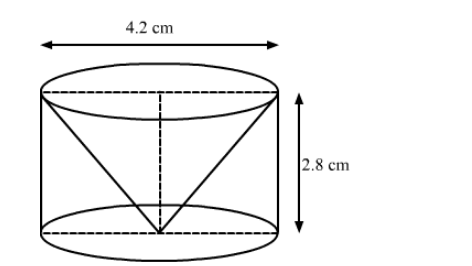# From a solid cylinder of height 2.8 cm and diameter 4.2 cm, a conical cavity of the same height and same diameter is hollowed out.`
Question:

From a solid cylinder of height 2.8 cm and diameter 4.2 cm, a conical cavity of the same height and same diameter is hollowed out. Find the total surface area of the remaining solid.

Solution:We have,

the height of the cone $=$ the height of the cylinder $=h=2.8 \mathrm{~cm}$ and

the radius of the base, $r=\frac{4.2}{2}=2.1 \mathrm{~cm}$

The slant height of the cone, $l=\sqrt{r^{2}+h^{2}}$

$=\sqrt{2.1^{2}+2.8^{2}}$

$=\sqrt{4.41+7.84}$

$=\sqrt{12.25}$

$=3.5 \mathrm{~cm}$

Now, the total surface area of the remaining solid = CSA of cylinder $+$ CSA of cone $+$ Area of a base

$=2 \pi r h+\pi r l+\pi r^{2}$

$=\pi r(2 h+l+r)$

$=\frac{22}{7} \times 2.1 \times(2 \times 2.8+3.5+2.1)$

$=22 \times 0.3 \times(5.6+5.6)$

$=6.6 \times 11.2$

$=73.92 \mathrm{~cm}^{2}$

So, the total surface area of the remaining solid is 73.92 cm2.